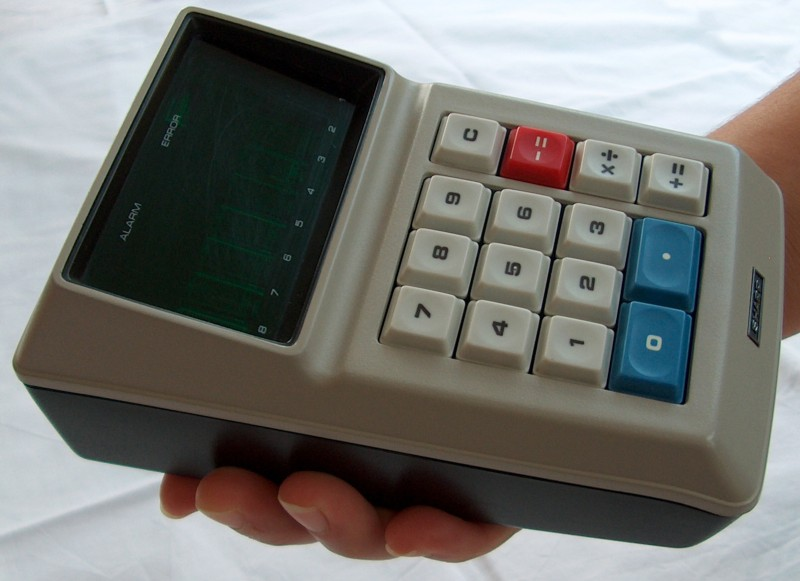# HP Forums

Full Version: Reverse engineering of the 1st Sharp calculator
You're currently viewing a stripped down version of our content. View the full version with proper formatting.
An interesting piece of history:

https://www.righto.com/2020/12/reverse-e...lator.htmlI wonder how did they use a combined multiply-divide button? Any ideas?
(12-27-2020 07:46 PM)celltx Wrote: [ -> ]I wonder how did they use a combined multiply-divide button? Any ideas?

I think... enter first value, press [×÷], then second value then [+=] for multiply or [-=] for divide.
(12-27-2020 08:53 PM)BruceH Wrote: [ -> ]
(12-27-2020 07:46 PM)celltx Wrote: [ -> ]I wonder how did they use a combined multiply-divide button? Any ideas?

I think... enter first value, press [×÷], then second value then [+=] for multiply or [-=] for divide.

That is correct.
"The QT-8D uses an unusual keyboard layout. Note the combined [X÷] key. Sharp used a novel means to save a key on the keyboard (thus saving the cost, as well as decreasing the real-estate needed for the keyboard) by making the [X÷] key serve both functions. The determination as to which function the user wishes is made by the user selecting either the [+=] key to generate the result for multiplication, and the [-=] key to cause division to occur. For example, to multiply, the user would enter the first number, press the [X÷] key, then enter the multiplier, then press the [+=] key for the answer. To divide, the user would enter the dividend, press the [X÷] key, enter the divisor, and press the [-=] key to calculate the answer."

http://bensene.com/sharpqt-8d.html
It could count as a very limited shift/prefix key. Like arc on the HP 35.

Pauli
Reference URL's
• HP Forums: https://www.hpmuseum.org/forum/index.php
• :